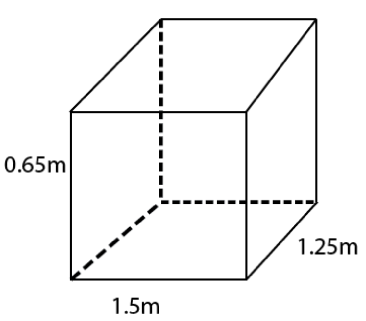Guru

# A plastic box 1.5 m long, 1.25 m wide and 65 cm deep, is to be made. It is to be open at the top. Ignoring the thickness of the plastic sheet, determine: (i)The area of the sheet required for making the box. (ii)The cost of sheet for it, if a sheet measuring 1m2 costs Rs. 20. Q.1

• 0

What is the easiest way for solving the question of class 9th ncert math of exercise 13.1   of math of Surface areas and Volumes chapter of question no.(1) Please help me for the best suggestion of this question A plastic box 1.5 m long, 1.25 m wide and 65 cm deep, is to be made. It is to be open at the top. Ignoring the thickness of the plastic sheet, determine: (i)The area of the sheet required for making the box. (ii)The cost of sheet for it, if a sheet measuring 1m2 costs Rs. 20.

Share

1.Given: length (l) of box = 1.5m

Breadth (b) of box = 1.25 m

Depth (h) of box = 0.65m

(i) Box is to be open at top

Area of sheet required.

= 2lh+2bh+lb

= [2×1.5×0.65+2×1.25×0.65+1.5×1.25]m2

= (1.95+1.625+1.875) m2 = 5.45 m2

(ii) Cost of sheet per m2 area = Rs.20.

Cost of sheet of 5.45 m2 area = Rs (5.45×20)

= Rs.109.

• 0